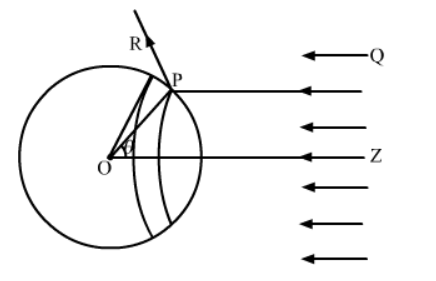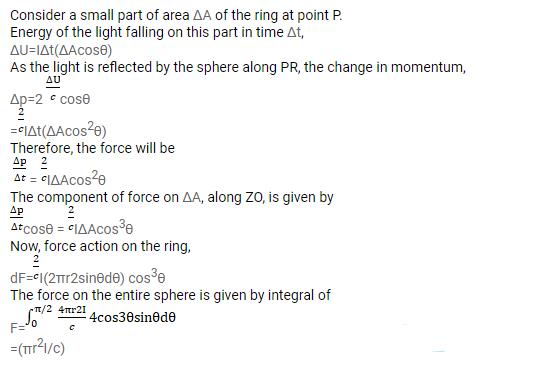# Consider the situation described in the previous problem.

Question:

Consider the situation described in the previous problem. Show that the force on the sphere due to the light falling on it is the same even if the sphere is not perfectly absorbing.

Solution:

Consider az sphere of centre $\mathrm{O}$ and radius OP. As shown in the figure, the radius OP of the sphere is making an angle $\theta$ with $\mathrm{OZ}$. Let us rotate the radius about $\mathrm{OZ}$ to get another circle on the sphere. The part of the sphere between the circle is a ring of area $2 \sqcap r^{2} \sin \theta d \theta$.